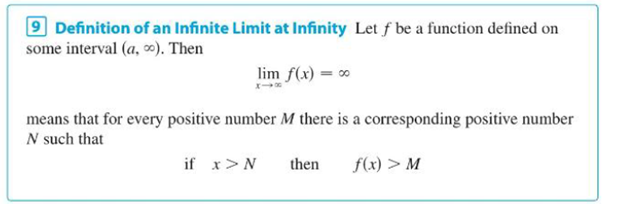Chapter 2.6, Problem 79E### Single Variable Calculus: Early Tr...

8th Edition
James Stewart
ISBN: 9781305270343

#### Solutions

Chapter
Section### Single Variable Calculus: Early Tr...

8th Edition
James Stewart
ISBN: 9781305270343
Textbook Problem

# Use Definition 9 to prove that lim x → ∞ e x = ∞ . Definition 9To determine

To prove: The value of limit of the function limxex=.

Explanation

Definition used:

Let f be a function defined on some interval (a,).

Then limxf(x)= means that for every positive number M there is a corresponding positive number N such that if x>N then f(x)>M.

Graph:

Proof:

Let the function f(x)=ex

Let M=ex1 some x1 is any real number.

M=ex1ln(M)=ln(ex1)ln(M)=x1

Choose N=ln(M) depends on M

### Still sussing out bartleby?

Check out a sample textbook solution.

See a sample solution

#### The Solution to Your Study Problems

Bartleby provides explanations to thousands of textbook problems written by our experts, many with advanced degrees!

Get Started

#### Find more solutions based on key concepts